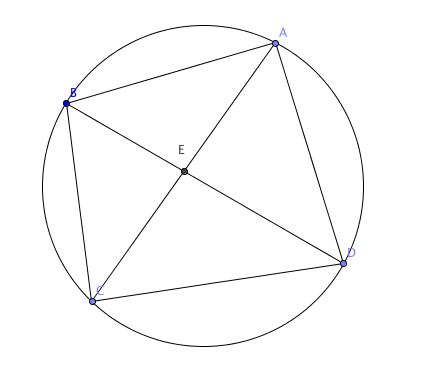# Triangles in a Circle

Probability Level 5

8 points are spaced around the boundary of a circle and all chords are drawn between them such that no three chords intersect in a point that is not on the circumference. How many different triangles can be seen in the resulting figure?

Details and assumptions

A triangle can be seen in the figure if all 3 sides are segments of the drawn chords.

As an explicit example, when there are $4$ points, we have 8 triangles, namely $ABC, BCD, CDA, DAB, EAB, EBC, ECD, EDA$, as seen below.×